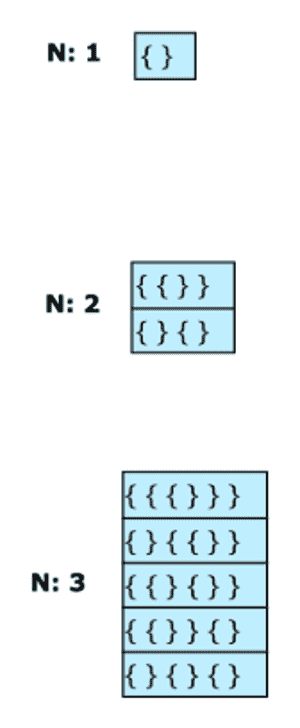# All Possible Braces

## Problem Statement

Print all braces combinations for a given value n so that they are balanced. Here are a few examples:• Recursion

## Try it yourself

vector<vector<char>> print_all_braces(int n) {  //TODO: Write - Your - Code  vector<vector<char>> result;  return result;}

## Solution

void print(vector<vector<char>> result){  for(int i = 0; i < result.size(); i++){    cout << "[ ";    for(int j = 0; j < result[i].size(); j++){      cout << result[i][j] << ", ";     }    cout << "]" << endl;  }}void print_all_braces_rec(    int n,    int left_count,    int right_count,    vector<char>& output, vector<vector<char>>& result) {  if (left_count == n && right_count == n) {    result.push_back(output);  }  if (left_count < n) {    output.push_back('{');    print_all_braces_rec(n, left_count + 1, right_count, output, result);    output.pop_back();  }  if (right_count < left_count) {    output.push_back('}');    print_all_braces_rec(n, left_count, right_count + 1, output, result);    output.pop_back();  }}vector<vector<char>> print_all_braces(int n) {  vector<vector<char>> result;  vector<char> output;  print_all_braces_rec(n, 0, 0, output, result);  return result;}int main() {  vector<vector<char>> result = print_all_braces(3);  print(result);}

## Solution Explanation

### Runtime complexity

The runtime complexity of this solution is exponential, $2^{n}$

### Memory complexity

The memory complexity of this solution is linear, O(n).

### Solution Breakdown

The solution is to maintain counts of left_braces and right_braces. The basic algorithm is as follows:​

left_braces count: 0
right_braces count: 0

if left_braces count is less than n:
add left_braces and recurse further
if right_braces count is less than left_braces count:
add right_braces and recurse further
stop recursing when left_braces and right_braces counts are both equal to n


Practice problems like this and many more by checking out our Grokking the Coding Interview: Patterns for Coding Questions course!

Learn in-demand tech skills in half the time